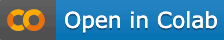### Import libraries​

``library(ChannelAttributionPro)``

``password="mypassword"``

``Data = read.csv("https://app.channelattribution.net/data/Data_ata.csv",sep=",")print(Data)``

### Define list of channels you are interested in performing attribution​

``channels=c("facebook_impressions","tv_impressions","google_adv_clicks",           "direct_searches","youtube_impressions","linkedin_impressions")``

### Define the global conversion rate for the use case or set it to None if not known​

``glob_conv_rate=NULL``

### Define the conversion rates, one for each channel if known, or set it to None otherwise​

``L_conv_rate=NULL``

### Define list of weights (between 0 and 1), one for each channel (suggestion: use weight=1 for clicks)​

``L_weight=list()L_weight["facebook_impressions"]=0.01L_weight["tv_impressions"]=0.01L_weight["google_adv_clicks"]=1L_weight["direct_searches"]=1L_weight["youtube_impressions"]=0.01L_weight["linkedin_impressions"]=0.01``

### Define the how many units of time are long the customer journeys on average for the use case considered​

``mean_cj_length=1``

### Define the adstock rates (between 0 and 1), one for each channels​

``L_ads_rate=list()L_ads_rate["facebook_impressions"]=0L_ads_rate["tv_impressions"]=0.40L_ads_rate["google_adv_clicks"]=0L_ads_rate["direct_searches"]=0L_ads_rate["youtube_impressions"]=0L_ads_rate["linkedin_impressions"]=0``

### Run the model​

#### we set nsim=1 for speed reasons but at least nsim=10 is suggested​

``res=hta_model(Data, var_time="week", var_conv="conversions", channels=channels, var_value=NULL,               glob_conv_rate=glob_conv_rate, L_conv_rate=L_conv_rate, L_weight=L_weight,               mean_cj_length=mean_cj_length, L_ads_rate=L_ads_rate, verbose=TRUE, nsim=1,               password=password)print(res)``